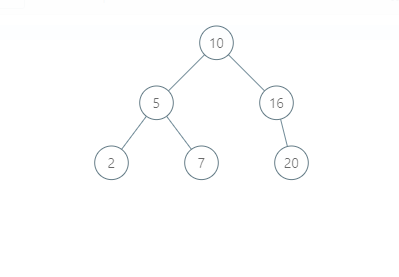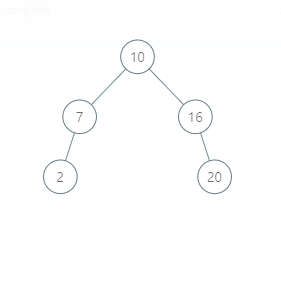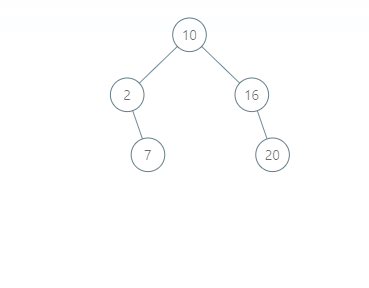# Delete Node In BST

Posted: 1 Dec, 2020
Difficulty: Moderate

## PROBLEM STATEMENT

#### A binary search tree (BST) is a binary tree data structure which has the following properties.

``````• The left subtree of a node contains only nodes with data less than the node’s data.
• The right subtree of a node contains only nodes with data greater than the node’s data.
• Both the left and right subtrees must also be binary search trees.
``````
##### Example:
``````For the given BST, if we want to delete the node with data 5 :
````````````The modified BST will be:
````````````Please notice that another valid answer is:
``````#### Note :

``````1. The node which we want to delete will always be present in the given tree.

2. If after deletion the tree becomes empty, print -1.
``````
##### Input Format:
``````The first line contains an integer 'T' which denotes the number of test cases.

The first line of each test case contains the elements of the tree in the level order form separated by a single space. If any node does not have a left or right child, -1 is used in its place. Refer to the example given below.

The second line of each test case contains the data of the node which we want to delete.
``````
##### Example:
``````Elements are in the level order form. The input consists of values of nodes separated by a single space in a single line. In case a node is null, we take -1 in its place.

For example, the input for the tree depicted in the below image would be :
1 2 3 4 -1 5 6 -1 7 -1 -1 -1 -1 -1 -1
``````##### Explanation :
``````Level 1 :
The root node of the tree is 1

Level 2 :
Left child of 1 = 2
Right child of 1 = 3

Level 3 :
Left child of 2 = 4
Right child of 2 = null (-1)
Left child of 3 = 5
Right child of 3 = 6

Level 4 :
Left child of 4 = null (-1)
Right child of 4 = 7
Left child of 5 = null (-1)
Right child of 5 = null (-1)
Left child of 6 = null (-1)
Right child of 6 = null (-1)

Level 5 :
Left child of 7 = null (-1)
Right child of 7 = null (-1)

1
2 3
4 -1 5 6
-1 7 -1 -1 -1 -1
-1 -1
``````
##### Note :
`````` 1. The first not-null node (of the previous level) is treated as the parent of the first two nodes of the current level. The second not-null node (of the previous level) is treated as the parent node for the next two nodes of the current level and so on.

2. The input ends when all nodes at the last level are null (-1).
``````
##### Output Format :
``````For each test case, print the inorder traversal of the modified Binary Search Tree.
``````
##### Note:
``````1. The inorder traversal of a binary tree is the traversal method in which for any node its left subtree is visited first, then the node itself, and then the right subtree.

2. You don't need to print the output, it has already been taken care of. Just implement the given function.
``````
##### Constraint :
``````1 <= T <= 100
1 <= N <= 5000
0 <= data <= 10^5

Where ‘N’ is the number of nodes in the binary search tree and ‘data' denotes data contained in the node of the binary search tree.

Time limit: 1 sec
``````Approach 1

When we delete a node, three possibilities arise:

1. Node to be deleted is a leaf node: Simply remove it from the tree.
2. Node to be deleted has only one child: Copy that child to the node and delete the child.
3. Node to be deleted has two children: Find the inorder successor of the node. Copy contents of the inorder successor to the node and delete the inorder successor.

The inorder successor of a node in BST is that node that will be visited immediately after the given node in the inorder traversal of the tree.

The Steps are as follows:

1. Recurse down the tree and find the node which needs to be deleted.
2. Once the node is found, we have to handle the below 4 cases.
1. Node doesn’t have a left or right subtree - return NULL.
2. Node only has the left subtree - return the left subtree.
3. Node only has the right subtree - return the right subtree.
4. Node has both left and right subtree:
1. Find the inorder successor of the node.
2. Copy contents of the inorder successor to the node.
3. Delete the inorder successor.